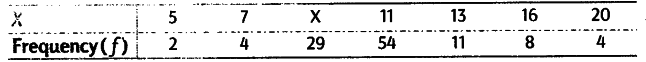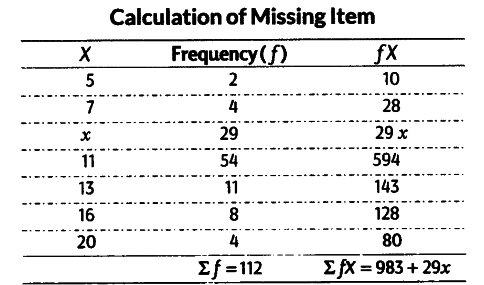# Find out the missing item x of the following distribution

Find out the missing item x of the following distribution, where arithmetic mean (X) is 11.37.29x = 290.44
x = 10.01 = 10
Hence, the missing value is 10.